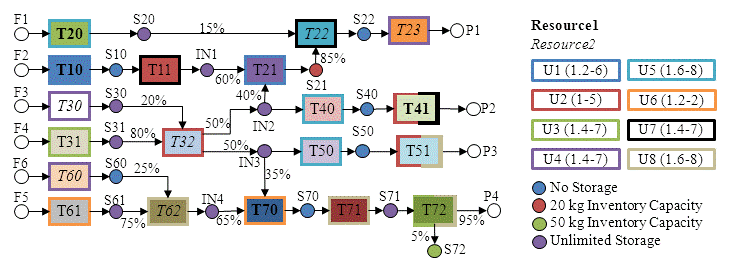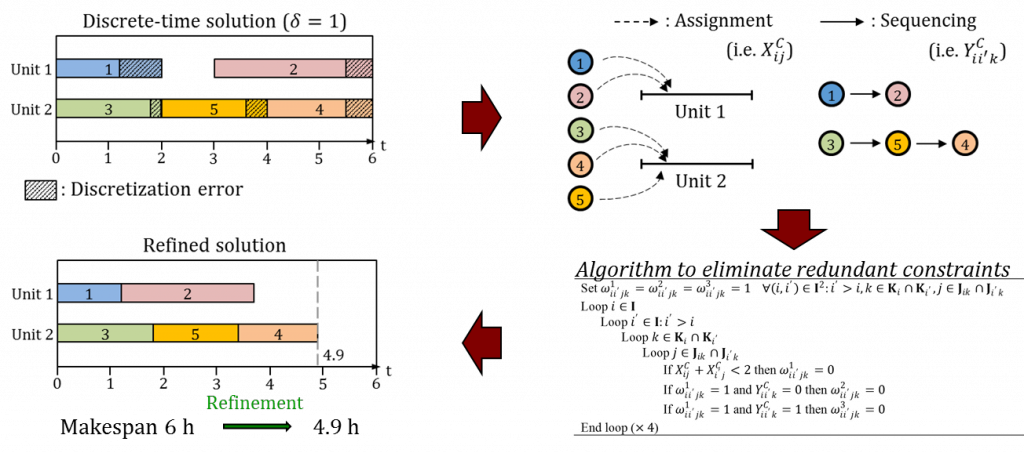University of Wisconsin – Madison

# Solution Methods

Despite advances in computer hardware and optimization software, the scheduling of chemical processes remains a hard problem. One of the goals of our research is to formulate mixed-integer programming (MIP) models for a wide range of scheduling problems. Since the solution of MIP scheduling models is computationally challenging, we aim to develop advanced solution methods:

1)  Tightening methods: Based on customer demand, process network characteristics and time related data, we calculate the total amount of material and number of batches each task must produce, as well as the number of times subsets of tasks can be executed in a feasible solution. Based on these amounts, we generate tightening constraints (Velez et al., 2013a; Merchan and Maravelias, 2016).

2)  Multi-grid discrete-time models: We formulate models based on separate grids for each task, processing unit, material, and utility. The proposed models have fewer binary variables and constraints than models based on a single and uniform time grid, thereby leading to faster solution times (Velez and Maravelias, 2015).

3)  Parallel branch-and-bound: We develop methods where (1) the full MIP is partitioned into many smaller MIPs by fixing or bounding the number of times each task runs, and (2) each smaller MIP is solved in parallel (Velez and Maravelias, 2013b).

4) Reformulations: We study extended reformulations where a new integer variable representing the total number of batches for each task is introduced. Branching on this new variable prevents the search from looking at many equivalent solutions and improves the bound on the optimal solution quickly (Velez and Maravelias, 2013c; Merchan et al., 2016).

5) Solution refinement method: We study a method that enable us to improve the quality of the solutions obtained from discrete-time formulations. We refine the discrete-time solution by re-optimizing the timing of events using a continuous-time model, while keeping the integer decisions fixed. Thus, hard instances can be solved faster with large discretization steps without any loss in accuracy (Merchan et al., 2016).

These methods can improve solution times by several orders of magnitude thereby allowing us to solve in minutes large-scale problems that were intractable (see Figures 1, 2 and 3).Figure 1. Example process network. ?ask outline color indicates which units can process it; font style indicates which resources are required. The color of each material indicates storage policy; unit capacities are given in parentheses.Figure 2. Solution to the instance shown in figure 1. A. Resource profile: total amount of each resource available, total amount in use, and cost. B. Inventory profile; feeds are received every 24 hours for the first 72 hours, and products have due dates every 24 hours starting at 48 hours. C. Gantt chart; tasks in the STN representation have the same color as the corresponding block in the Gantt chart.Figure 3. An illustration of solution refinement method. The objective value of discrete-time solution obtained using large discretization step of 1 hour is improved from makespan of 6 hours to 4.9 hours after the refinement, which proved to be the optimal solution when fine discretization of 0.1 hour is used.

Reference:

Merchan, A. F., Lee, H. and Maravelias, C. T. (2016) Discrete-Time Mixed-Integer Programming Models and Solution Methods for Production Scheduling in Multistage Facilities. Computers and Chemical Engineering.

Merchan, A. F. and Maravelias, C. T. (2016) Preprocessing and tightening methods for time-indexed MIP chemical production scheduling models. Computers & Chemical Engineering. 84, 516-535.

Velez, S., Sundaramoorthy, A. and Maravelias, C. T. (2013a) Valid Inequalities Based on Demand Propagation for Chemical Production Scheduling MIP Models. Aiche Journal. 59, 872-887.

Velez, S. and Maravelias, C. T. (2013b) A branch-and-bound algorithm for the solution of chemical production scheduling MIP models using parallel computing. Computers & Chemical Engineering. 55, 28-39.

Velez, S. and Maravelias, C. T. (2013c) Reformulations and Branching Methods for Mixed-Integer Programming Chemical Production Scheduling Models. Industrial & Engineering Chemistry Research. 52, 3832-3841.

Velez, S. and Maravelias, C. T. (2015) Theoretical framework for formulating MIP scheduling models with multiple and non-uniform discrete-time grids. Computers & Chemical Engineering. 72, 233-254.